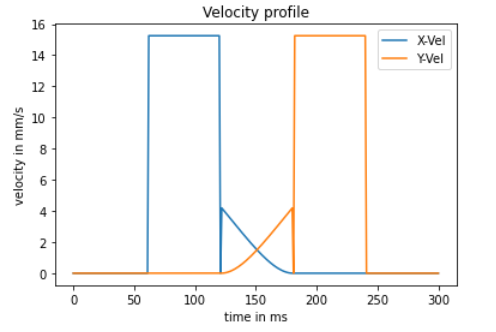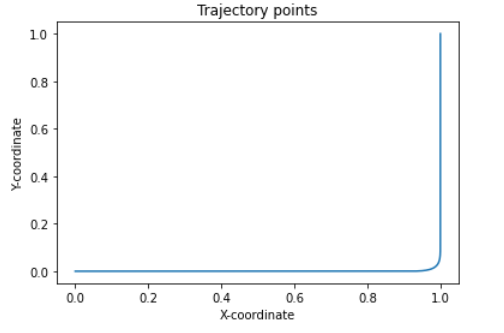# How to generate smooth velocity profile?

Hi OpenCN developer,

I tried to execute trajectory planning using generated c++ files in <opencn_home>/agency/usr/matlab/x86/matlab/generated. I was trying to generate trajectory points by following the approach given in the file: <opencn_home>/agency/usr/rs274ngc/main.cpp, basic lines are:

ocn::FeedoptDefaultConfig(&cfg);
ocn::ConfigSetSource(&cfg, “Test.ngc”, {1, 8});
ocn::FeedoptContext ctx;
InitFeedoptPlan(cfg, &ctx);
bool optimized = false;
ocn::CurvStruct Curv;
while (ctx.op != ocn::Fopt::Fopt_Opt) {
ocn::FeedoptPlan(&ctx, &optimized, &Curv);
if (optimized) {
ctx.go_next = true;
optimized = false;
}
}
for(int i = 0; i < ctx.q_split.size(); i++) {
ocn::CurvStruct tmp_curv;
ctx.q_split.get(i + 1, &tmp_curv);
}

function add_curv, gets a spline object from ctx->q_splines and calls EvalPosition to compute the trajectory points.

void add_curv(const ocn::CurvStruct *c,const ocn::FeedoptContext *ctx, FILE *fptr, FILE *fptrY) {
ocn::CurvStruct Spline;
ctx->q_splines.get(c->sp_index, &Spline);
const int N = 60;
for(int i = 0; i < N; i++) {
ocn::EvalPosition(c, &Spline, (float)i/(N - 1), r0D);
//code to write r0D (x-coordinate), and r0D (y-coordinate) in file pointer *fptr and *fptrY respectively
//
}

r0D is static array of double, and I am writing corresponding 8 bytes of double to the opened file pointers.

My Test.ngc looks as follow:
G17 G21 G40 G49
G64 P2.0
(G61.1)
G00 X0 Y0 Z0
G1 X1 Y0 Z0
G1 X1 Y1 Z0
M2

My FeedoptDefaultConfig is as follow:
cfg->NDiscr = 140;
cfg->NBreak = 60;
cfg->UseDynamicBreakpoints = false;
cfg->UseLinearBreakpoints = true;
cfg->DynamicBreakpointsDistance = 0.1;
cfg->NHorz = 5;
cfg->vmax = 15.0;
cfg->amax = 20.0;
cfg->jmax = 2000;
cfg->amax = 20.0;
cfg->jmax = 2000;
cfg->amax = 20.0;
cfg->jmax = 2000;
cfg->SplineDegree = 4;
cfg->CutOff = 0.1;
cfg->LSplit = 3.0;
cfg->LThreshold = 0.3;
cfg->CuspThreshold = 45.0;
cfg->v_0 = 0.1;
cfg->at_0 = 0.0;
cfg->v_1 = 0.1;
cfg->at_1 = 0.0;
// cfg->dt = 0.0001;
cfg->dt = 0.001;
cfg->ZeroStartAccLimit = 0.01;
cfg->ZeroStartJerkLimit = 1.0;
cfg->ZeroStartVelLimit = 0.5;
std::memset(&cfg->source, 0, 1024U * sizeof(char));
cfg->DebugCutZero = false;
cfg->Compressing.Skip = false;
cfg->Compressing.ColTolDeg = 5.0;
cfg->CollTolDeg = 1.0E-6;
cfg->NGridLengthSpline = 10.0;

Though generated trajectory points seem to be correct according to the graph, but the velocity profile is not smooth leading to discontinuous acceleration, which leads to infinite jerk.

I am attaching plot of my velocity profile for reference.Thank you

I am also attaching the plot of my trajectory points for referencedifficult to say what is wrong here.
Of course the velocity profile must be smooth, respecting the acceleration and jerk constraints.

On Matlab side we have a script called ValidateOpenCN.
It sweeps all .ngc files in a directory, calculates the whole computation chain, and checks if the constraints are respected. It also checks time optimality by looking if at all time at least one constraint is active. This is on Matlab side.

The machine we use is running for at least 2 years on OpenCN.
We checked the generated setpoint values using the OpenCN component “sampler”.
This allows you to store all samples of one or more signals (=HAL pins) into an ASCII file.

I advise against changing the C++ generated filesSorry I forgot to mention that I have not changed anything in generated C++ files.

Ok, but I still don’t understand how you use OpenCN.
If you use it directly on the machine, I recommend the utilization of the external GUI.

If you use it for test purposes in order to check the path planning, I recommend to use it directly from Matlab. You can in principle use the script ValidateOpenCN.m

Hello, I tried to run the script ValidateOpenCN.m, but my script was not able to run because it could not recognized function “bspline_create_mex”. Are there any particular steps/settings, which I need to do in order to run this script? If yes then can you please help me with the same, or is there a document which I can follow to get this working?

I am using Matlab2020a on windows 10 environment. I also have added path of the <opencn_home>\agency\usr\matlab in my matlab environment.

I saw another thread, where a user was trying to run Matlab Trajectory Planning script. Should I post the question there?

you need to run Matlab on Linux !

While .m files are compatible on both operating system, mex files (=binaries) are NOT.
The mex files are compiled parts of C/C++ code, e.g. the RS274 interpreter of .ngc files, numerical libraries (e.g. for B-splines, CLP solver, etc).